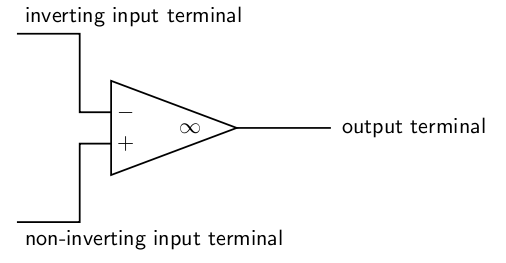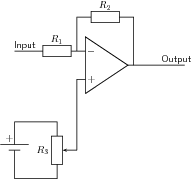# 13.2 Active circuit elements  (Page 7/7)

 Page 7 / 7

## The operational amplifier

The operational amplifier is a special kind of voltage amplifier which is made from a handful of bipolar or field effect transistors. Operational amplifiers are usually called op-amps for short. They are used extensively in all kinds of audio equipment (amplifiers, mixers and so on) and in instrumentation. They also have many other uses in other circuits - for example comparing voltages from sensors.

Operational amplifiers are supplied on Integrated Circuits (I.C.s). The most famous operational amplifier I.C. is numbered 741 and contains a single operational amplifier on an integrated circuit (chip') with eight terminals. Other varieties can be bought, and you can get a single integrated circuit with two or four 741'-type operational amplifiers on it.

The symbol for an op-amp is shown in [link] . The operational amplifier has two input terminals and one output terminal. The voltage of the output terminal is proportional to the difference in voltage between the two input terminals. The output terminal is on the right (at the sharp point of the triangle). The two input terminals are drawn on the left. One input terminal (labelled with a $+$ on diagrams) is called the non-inverting input . The other input terminal (labelled $-$ ) is called the inverting input . The labels $+$ and $-$ have nothing to do with the way in which the operational amplifier is connected to the power supply. Operational amplifiers must be connected to the power supply, but this is taken for granted when circuit diagrams are drawn, and these connections are not shown on circuit diagrams. Usually, when drawing electronic circuits, 0V' is taken to mean the negative terminal of the power supply. This is not the case with op-amps. For an op-amp, 0V' refers to the voltage midway between the $+$ and $-$ of the supply.Circuit symbol for an operational amplifier. The amplifier must also be connectd to the + and - terminals of the power supply. These connections are taken for granted and not shown.

The output voltage of the amplifier ${V}_{out}$ is given by the formula ${V}_{out}=A\left({V}_{+},-,{V}_{-}\right)$ where $A$ is a constant called the open loop gain , and ${V}_{+}$ and ${V}_{-}$ are the voltages of the two input terminals. That said, the output voltage can not be less than the voltage of the negative terminal of the battery supplying it or higher than the positive terminal of the battery supplying it. You will notice that ${V}_{out}$ is positive if ${V}_{+}>{V}_{-}$ and negative if ${V}_{+}<{V}_{-}$ . This is why the $-$ input is called the inverting input: raising its voltage causes the output voltage to drop .

The input resistance of an operational amplifier is very high. This means that very little current flows into the input terminals during operation.

If all of the transistors in the operational amplifier were identical then the output voltage would be zero if the two inputs were at equal voltages. In practice this is not quite the case, and for sensitive work a trimming potentiometer is connected. This is adjusted until the op-amp is zeroed correctly.

Simple operational amplifiers require the trimming potentiometer to be built into the circuit containing them, and an example is shown in [link] . Other operational amplifier designs incorporate separate terminals for the trimming potentiometer. These special terminals are labelled offset on the manufacturer's diagram. The exact method of connecting the potentiometer to the offset terminals can depend on the design of the operational amplifier, and you need to refer to the manufacturer's data sheet for details of which potentiometer to use and how to connect it.

For most commercially produced operational amplifiers (known as op-amps for short), the open loop gain $A$ is very large and does not stay constant. Values of 100 000 are typical. Usually a designer would want an amplifier with a stable gain of smaller value, and builds the operational amplifier into a circuit like the one in [link] .An inverting amplifier built using an operational amplifier. The connections from battery to operational amplifier are not shown. The output voltage V o u t = - R 2 V i n / R 1 , as explained in the text. The potentiometer R 3 is a trimming potentiometer. To set it, the input is connected to zero volts. The trimming potentiometer is then adjusted until V o u t = 0 . In all operational amplifier circuits, zero volts is midway between the + and - of the supply.

## Calculating the gain of the amplifier in [link] .

1. The input resistance of the operational amplifier is very high. This means that very little current flows into the inverting input of the op-amp. Accordingly, the current through resistor ${R}_{1}$ must be almost the same as the current through resistor ${R}_{2}$ . This means that the ratio of the voltage across ${R}_{1}$ to the voltage across ${R}_{2}$ is the same as the ratio of the two resistances.
2. The open loop gain $A$ of the op-amp is very high. Assuming that the output voltage is less than a few volts, this means that the two input terminals must be at very similar voltages. We shall assume that they are at the same voltage.
3. We want the output voltage to be zero if the input voltage is zero. Assuming that the transistors within the op-amp are very similar, the output voltage will only be zero for zero input voltage if ${V}_{+}$ is very close to zero. We shall assume that ${V}_{+}=0$ when the trimming potentiometer is correctly adjusted.
4. It follows from the last two statements that ${V}_{-}\approx 0$ , and we shall assume that it is zero.
5. With these assumptions, the voltage across ${R}_{2}$ is the same as ${V}_{out}$ , and the voltage across ${R}_{1}$ is the same as ${V}_{in}$ . Since both resistors carry the same current (as noted in point 1), we may say that the magnitude of ${V}_{out}/{V}_{in}={R}_{2}/{R}_{1}$ . However, if ${V}_{in}$ is negative, then ${V}_{out}$ will be positive. Therefore it is customary to write the gain of this circuit as ${V}_{out}/{V}_{in}=-{R}_{2}/{R}_{1}$ .

## Operational amplifiers

1. What are operational amplifiers used for?
2. Draw a simple diagram of an operational amplifier and label its terminals.
3. Why is a trimming potentiometer needed when using an op-amp?

what makes an atom to take part in a chemical reaction
what is an element
Mwila
what are molecules
what are molecules
A group of two atoms tha the form smallest identifiable unit into which a pure substance.
El
what is matter
it's a topic in physics
Thabo
Anything that occupies space and has mass
Lisoga
what is the catalyst used in esterification reaction
I think is sulfuric acid
Selby
what is equilibrium
It is a condition of a system when neither its state of motion nor its internal energy state tends to change with time. ... For a single particle, equilibrium arises if the vector sum of all forces acting upon the particle is zero.
susan
it is a dynamic change when the forward reaction is equals to the reverse reaction rate
Frank
what does organic mean
it means "from living thing"
Maqaqatu
What is a successful collision?
It's a collision that results to a reaction
Tshepo
what is the factor and reaction rate of 20%HCL and 5%HCL
concentration and 0.05M/s
Tokozan
how you reaching with apartment depends on what each of you have
how with 4looseyellowprotons in your left hand stars are mostly hydrogen so this is your fuel you will also need one six so did die
Edelita
besides the concentration what are the two conditions for the hydrogen half cell to function under standard conditions
forces of non-concervative
What is the following process is likely to involve carbon burning?
Combustion
Luyanda
Which Statement is part of the cell theory?
What is the second formal statement in the cell theory
Hananiya
🤔
Bontle
what is meant by ohmic
when someone is referred to as ohmic, it basically means that it obeys ohm's law
Tanaka
*something
Tanaka

#### Get Jobilize Job Search Mobile App in your pocket Now!By Janet ForresterBy Stephen VoronByBy Stephen VoronBy OpenStaxBy Yacoub JayoghliBy JavaChamp TeamBy OpenStaxBy OpenStaxBy Cath Yu